## Resource of the Week: Reflective symmetry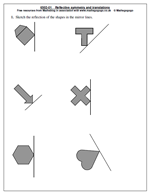There is quite a lot of work done in Year 6 on symmetry and the subject often comes up in the Key Stage 2 SATs.

Some of this involves important vocabulary and by the end of year 6 children should know and be able to read, write and use the following quite tricky words:

Mirror line,  line of symmetry,  line symmetry,  symmetrical,  reflect,  reflection,  translation, axis of symmetry, reflective symmetry.

Also they should be able to test for symmetry using a mirror and by folding.

Children are expected to sketch the reflection of a simple shape in a mirror line where none or only some of the edges of the shape are parallel or perpendicular to the mirror line.

This might seem easy, but actually prove problematic to many children. A small mirror or tracing paper can be a great help with this.

Year 6 maths worksheet: reflective symmetry

## Year 4 maths worksheet: Tenths of rectangles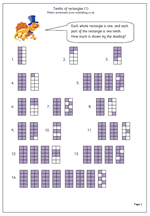A good way of working with decimals is to use shapes which are divided into ten equal parts. The shape is one whole unit and each part is one tenth, which can be written as 0.1.

This page has a selection of shapes and asks the child to write down the amount that has been shaded. Where all parts of a shape have been shaded this counts as ten tenths, or one whole one.

Whilst printed pages nearly always show the decimal point situated on the line most schools show it as half way up the line: the most important thing is that it shows up clearly.

This page can be found in our Year 4 maths worksheets in the Counting and Number category.

Tenths of rectangles (1)

## Year 1 maths vocabulary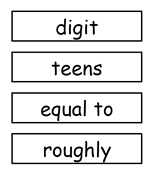If a child does not respond to a mathematical question it may be because they do not understand the words or instructions used. Another difficulty can arise when a mathematical term has a different meaning in everyday English eg ‘table’.

In the Year 1 maths category there is an excellent list of the vocabulary children need to understand and use in year 1. For example, the following list has the new words that they should come across in school during year 1 to do with calculating and solving problems.

Words to do with calculating and solving problems:

plus                                     near double

how much more is…?     how much less is…?

subtract                             minus

half                                     halve

equals                               sign

number sentence

operation

There are similar lists for calculating, measures and position. These words can be printed larger so that they can be used as ‘flash cards’ or displayed on the wall.

Go to the Year 1 vocabulary lists.

## Year 3 Mental Arithmetic: Sets 5 and 6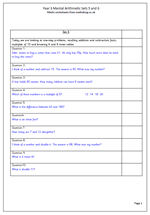This week I have published the next two sets of mental arithmetic questions for Year 3. They concentrate on one-step problems, recalling addition and subtraction facts up to 20,adding and subtracting multiples of ten and knowing 5 and 4 times tables.

There are several ways these questions can be used and how children answer them. It is a good idea sometimes to use a set of digit cards, so children can hold up the answer. Number fans serve a similar purpose. Sometimes you might want the answers to be called out, or to be written down. The question sheets can be used for this purpose.

Y3 mental arithmetic sets 5 and 6

## Year 5 maths: Written multiplication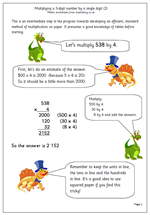Here we have a second page of questions  which are an intermediate step in the progress towards developing an efficient, standard written method of multiplication. It presumes a good knowledge of tables before starting.

This method breaks the task down into four parts. For example: when multiplying 538 by 4, the four parts are:

1. multiply 500 by 4

2. Multiply 30 by 4

3. Multiply 8 by 4.

Whilst this is a good method I prefer to work in the reverse order of starting with the units, then the tens and finally the hundreds, although if the child understanding what is happening it makes little real difference.

Multiply a 3 digit number by a 1 digit number (2)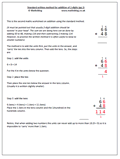One of the most popular parts of the site for parents is our Free Maths Worksheets: Four Rules pages on written addition.

Many parents think that the methods they were taught at school (and for many this would be in the mid 1990s) are different from today. This is only partly true. Since the onset of the Numeracy Strategy and later the Primary Framework for Maths the emphasis has been on preparing children properly so that they understand the written methods they use. So there is a lot of preparation work and methods often called, ‘Moving towards the standard written method’ that may not be familiar to parents, but the end result is pretty much the same. It is these intermediate stages which some parents find puzzling.

The standard written method is to lay out the sum vertically, with the numbers to be added under each other. The units are added first, then the tens and so on. We have several worksheets which explain this method in full.

Go to Written Methods of Addition

## Year 6 Maths Worksheet: Equivalent fractions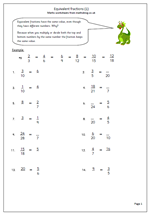Understanding equivalent fractions is at the heart of understanding how fractions work. Much work needs to be done in earlier years to show that fractions such as 1/2 and 2/4 have the same value. This is usually done by colouring or shading parts of rectangles, circles.

Two fractions which are equal in value we say are equivalent.

2/5 is equivalent to 4/10 which is equivalent to 40/100.

If we start with a fraction and multiply both the numerator (top number) and the denominator (bottom number) by the same number the fraction will remain with the same value. The same is true with division, but not addition or subtraction.

This makes multiplying fractions much easier than adding or subtracting them.

Equivalent fractions (pg 1)

## Year 4 worksheets: Division with remainders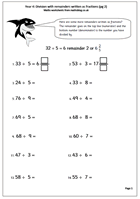Division nearly always requires carrying out more than one process, which makes it harder for children to process. A good knowledge of tables is also needed to complete division questions successfully, and this is especially true when there are remainders.

For example: to find the answer to 37 divided by 5: ask how many fives are in 37. This is where the knowledge of tables comes in, as 5 x 7 makes 35.

Then a subtraction take 35 from 37 to leave 2. There is a remainder of 2. (The remainder can never be bigger than the number you are dividing by.)

This remainder can be converted to a fraction. The 2 is the numerator (top number) and the denominator (bottom number) is the number you are dividing by, in this case 5. So the fraction left is 2/5.

This is a much neater finish to the question as the whole number is divided completely, with no messy remainders. Some of the fractions could be cancelled down to their simplest form, although this is not essential at this stage.

Division with fraction remainders (pg 2)

## Year 3 Mental Arithmetic Sets 3 and 4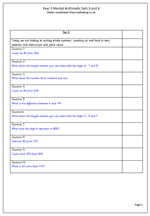Two sets of mental arithmetic questions suitable for Year 2. These sets of 10 questions cover counting on and back in tens, addition, subtraction and place value.

I have been asked for more in the way of mental arithmetic questions on many occasions and as the weeks go by this should build into a really useful resource. I am also trying to match the questions to the Primary Framework for Mathematics Planning, so this would be especially relevant for the first couple of weeks of the Autumn term.

Year 3_Mental arithmetic (3 and 4)

## Maths worksheets: Division of money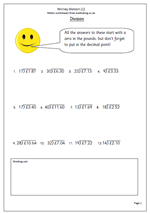There are not many questions on this maths worksheet but they are quite tricky and a good knowledge of tables and how to do long division is needed.

Each question has an amount of money to be divided by a 1 or 2-digit number. In all cases the answer wil have a zero in the pounds column.

As well as the long division process there are several key things to remember when doing these, including: